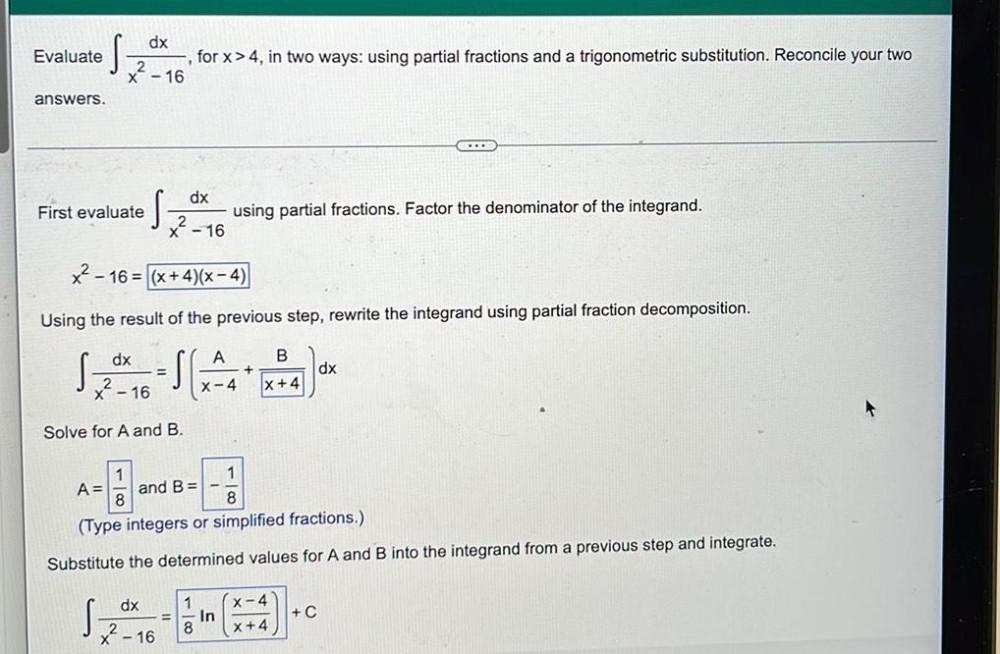Question:

# Evaluate s dx , for x>4, in two ways: using partial fractions and a trigonometric substitution. Reconcile your two 2 x - 16 answEvaluate s dx , for x>4, in two ways: using partial fractions and a trigonometric substitution. Reconcile your two 2 x - 16 answers. First evaluate S dx x? - 16 using partial fractions. Factor the denominator of the integrand. x2 - 16 = (x+4)(x-4) Using the result of the previous step, rewrite the integrand using partial fraction decomposition. dx A B + dx X-4 |x+4| x - 16 Solve for A and B. 1 A= and B= 8 8 (Type integers or simplified fractions.) Substitute the determined values for A and B into the integrand from a previous step and integrate. dx X-4 s 1 In 8 + C X? - 16 X+4